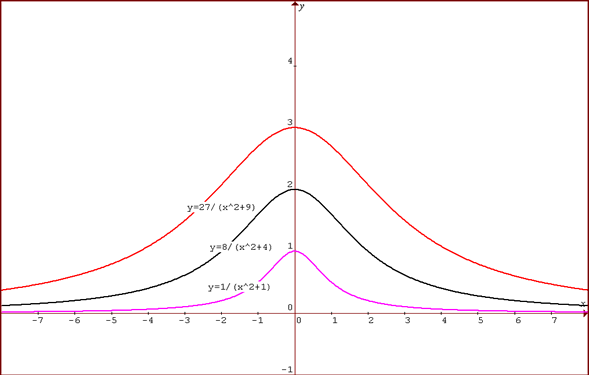#### You may also like### How Many Solutions?

Find all the solutions to the this equation.### Quartics

Investigate the graphs of y = [1 + (x - t)^2][1 + (x + t^)2] as the parameter t varies.### Power Up

Show without recourse to any calculating aid that 7^{1/2} + 7^{1/3} + 7^{1/4} < 7 and 4^{1/2} + 4^{1/3} + 4^{1/4} > 4 . Sketch the graph of f(x) = x^{1/2} + x^{1/3} + x^{1/4} -x

# Witch of Agnesi

##### Age 16 to 18Challenge Level

Sanjeet sent us this graph, showing the three curves on the same graph:For different values of $a$ the graphs have the equation: $$y = {a^3\over (x^2+a^2)}$$ Differentiating $${dy\over dx} = - {2a^3x\over (x^2+a^2)^2}.$$ So the critical value is at $(0,a)$. Near this point, for small negative $x$ the gradient is positive and the function increasing and for small positive $x$ the gradient is negative and the function decreasing so this is a maximum point.

The value of $y$ is always positive so the entire graph lies above the $x$ axis and the graph is symmetrical about $x=0$ because $f(-x)=f(x)$.

For large $x$ the value of $y$ tends to zero so the line $y=0$ is an asymptote.